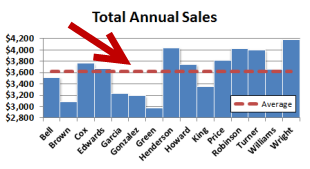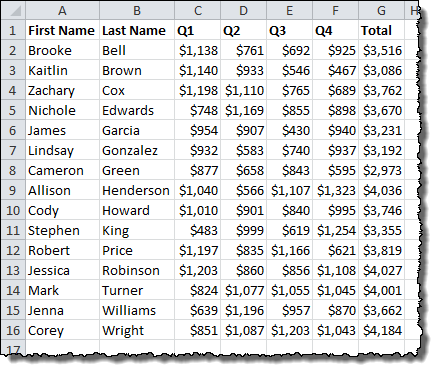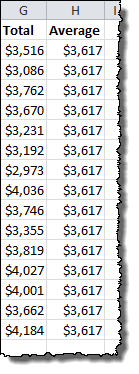# How To Add an Average Value Line to a Bar ChartWhen you are comparing values in a bar chart, it is useful to have some idea of what the average value looks like relative to the data set. By default, however, Excel’s graphs show all data using the same type of bar or line. In this quick tutorial, we’ll walk through how to add an Average Value line to a vertical bar chart by adding an aggregate statistic (Average) to a data set and changing a series chart type.

## Updating the Data Set

In our example, we are starting with a basic set of sales figures broken out by employee. We have quarterly figures and an annual total.We want to display the average annual sales for the entire group in our chart, so we need to calculate a new column that we’ll call Average. Since this is going to be a line, we need every cell in the column to have the same average calculation, so we’ll lock the cell references before we drag down. The AVERAGE formula will be as follows:

`=AVERAGE(\$G\$2:\$G\$16)`

The column will look like this: